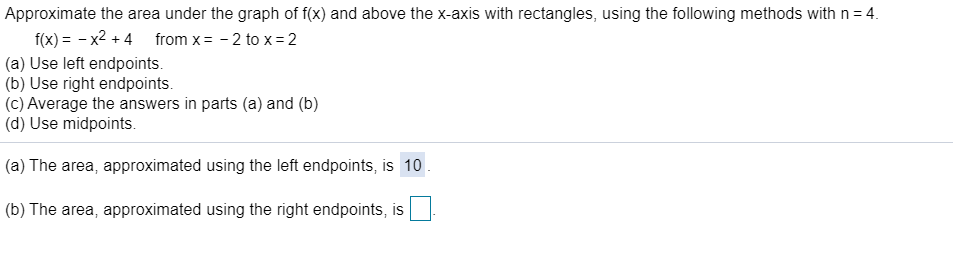# Approximate the area under the graph of f(x) and above the x-axis with rectangles, using the following methods with n 4.f(x)x24(a) Use left endpoints.(b) Use right endpoints.(c) Average the answers in parts (a) and (b)(d) Use midpoints.from x2 to x 2(a) The area, approximated using the left endpoints, is 10(b) The area, approximated using the right endpoints, is

Question
2 viewshelp_outlineImage TranscriptioncloseApproximate the area under the graph of f(x) and above the x-axis with rectangles, using the following methods with n 4. f(x)x24 (a) Use left endpoints. (b) Use right endpoints. (c) Average the answers in parts (a) and (b) (d) Use midpoints. from x 2 to x 2 (a) The area, approximated using the left endpoints, is 10 (b) The area, approximated using the right endpoints, is fullscreen
check_circle

Step 1

Since we only answer up to 3 sub-parts, we’ll answer the first 3. Please resubmit the question and specify the other subparts (up to 3) you’d like answered

Step 2

Given, function is

Step 3

Reimann sum...

### Want to see the full answer?

See Solution

#### Want to see this answer and more?

Solutions are written by subject experts who are available 24/7. Questions are typically answered within 1 hour.*

See Solution
*Response times may vary by subject and question.
Tagged in

### Other